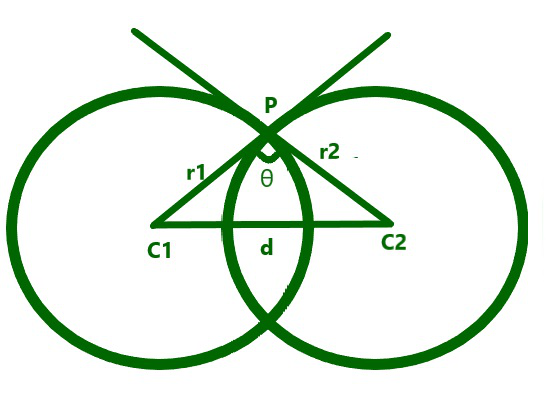GFG App
Open AppBrowser
Continue

# Angle of intersection of two circles having their centers D distance apart

Given two positive integers R1 and R2 representing the radius of two intersecting circles having a distance D between their centers, the task is to find the cosine of the angle of intersection between the two circles.

Examples:

Input: R1 = 3, R2 = 4, D = 5
Output: 0

Input: R1 = 7, R2 = 3, D = 6
Output: 0.52381

Approach: The given problem can be solved by using the Geometric Algorithm as illustrated below:From the above image and using the Pythagoras Theorem, the cosine of the angle of intersection of the two circles can be found using the formula:Below is the implementation of the above approach:

## C++

 // C++ program for the above approach #include  using namespace std;   // Function to find the cosine of the // angle of the intersection of two // circles with radius R1 and R2 float angle(float R1, float R2, float D) {     float ans = (R1 * R1 + R2 * R2 - D * D)                 / (2 * R1 * R2);       // Return the cosine of the angle     return ans; }   // Driver Code int main() {     float R1 = 3, R2 = 4;     float D = 5;     cout << angle(R1, R2, D);       return 0; }

## Java

 // Java program for the above approach  import java.io.*;   class GFG{       // Function to find the cosine of the // angle of the intersection of two // circles with radius R1 and R2 static float angle(float R1, float R2, float D) {     float ans = (R1 * R1 + R2 * R2 - D * D) /                 (2 * R1 * R2);                      // Return the cosine of the angle     return ans; }   // Driver Code public static void main (String[] args) {     float R1 = 3, R2 = 4;     float D = 5;           System.out.println(angle(R1, R2, D)); } }   // This code is contributed by Ankita saini

## Python3

 # Python3 program for the above approach   # Function to find the cosine of the # angle of the intersection of two # circles with radius R1 and R2 def angle(R1, R2, D):           ans = ((R1 * R1 + R2 * R2 - D * D) /             (2 * R1 * R2))       # Return the cosine of the angle     return ans   # Driver Code if __name__ == '__main__':           R1 = 3     R2 = 4     D = 5           print(angle(R1, R2, D))       # This code is contributed by ipg2016107

## C#

 // C# program for the above approach  using System;   class GFG{       // Function to find the cosine of the // angle of the intersection of two // circles with radius R1 and R2 static float angle(float R1, float R2, float D) {     float ans = (R1 * R1 + R2 * R2 - D * D) /                 (2 * R1 * R2);                      // Return the cosine of the angle     return ans; }   // Driver Code public static void Main(string[] args) {     float R1 = 3, R2 = 4;     float D = 5;           Console.Write(angle(R1, R2, D)); } }   // This code is contributed by rutvik_56.

## Javascript

 

Output:

0

Time Complexity: O(1)
Auxiliary Space: O(1)

My Personal Notes arrow_drop_up